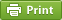Werner's Blog — Opinion, Analysis, Commentary
Triangular Distribution Estimation

Triangular distributions are often used in empirical work as rough-and-ready approximations of more complicated distributions when the objective is to simulate data and draw random numbers that are "well-behaved". Drawing from a normal distribution can generate numbers that are in the long tails of the distribution. Triangular distributions have the property that they are bounded. Specifically, they have a lower and upper boundary (L and H), and a mode (M) at which the probability density function peaks. The triangular distribution has the probability density function $\phi(x)=\left\{\begin{array}{ll} 2(x-L)/[(H-L)(M-L)]&\mathrm{if}\ L\le x< M\\ 2/(H-L)&\mathrm{if}\ x=M\\ 2(H-x)/[(H-L)(H-M)]&\mathrm{if}\ M< x\le H \end{array}\right.$ and the cumulative distribution function $\Phi(x)=\left\{\begin{array}{ll} (x-L)^2/[(H-L)(M-L)]&\mathrm{if}\ L\le x\le M\\ 1-(H-x)^2/[(H-L)(H-M)]&\mathrm{if}\ M< x\le H \end{array}\right.$ But how can one quickly estimate the three parameters H, M, and L when one needs to simulate this distribution?

Given a set of data points $$x_i$$, the quickest way for fixing the three parameters involves $$L^\ast=\min\{x_i\}$$, $$H^\ast=\max\{x_i\}$$, and given the mean $$\mu=(L+M+H)/3$$ of the distribution, the mode can be calculated from $$M^\ast=3\cdot\mu-H^\ast-L^\ast$$. Another method involves the three moments of the distribution: mean, variance, and skewness. These are three equations that can be solved for the three parameters H, M, and L. Unfortunately, it requires non-linear equation solving: no computational challenge, but not very elegant.

Thinking of elegant solutions for estimation, I found a way to estimate the three parameters from four quantiles of the empirical distribution. The diagram below shows the four quantile points $$Q_r$$ to be used.

The quantiles on the left and right are given by $\begin{array}{lll} Q_{1/16}&=&L+(1/4)\sqrt{(H-L)(M-L)}\\ Q_{1/4}&=&L+(1/2)\sqrt{(H-L)(M-L)} \\ Q_{3/4}&=&H-(1/2)\sqrt{(H-L)(H-M)} \\ Q_{15/16}&=&H-(1/4)\sqrt{(H-L)(H-M)} \end{array}$ and can be determined easily from the raw data $$\{x_i\}$$. The first two equations can be solved for L because the square root expressions are the same in both and can be substituted out. The same method works for the pair of last two equations for determining H. To determine M, we need to define two auxiliary variables that capture the difference between the quantiles on the left and right, respectively. The square of these two differences become auxiliary variables $$u$$ and $$v$$, respectively. $\begin{array}{lll} u &=& (Q_{1/4}-Q_{1/16})^2\\ v &=& (Q_{15/16}-Q_{3/4})^2\\ L^\ast&=&2\cdot Q_{1/16}-Q_{1/4}\\ H^\ast&=&2\cdot Q_{15/16}-Q_{3/4}\\ M^\ast&=&\displaystyle\frac{u\cdot H^\ast+v\cdot L^\ast}{u+v} \end{array}$

The formula for $$M^\ast$$ can be derived by noting that $$M=L+\alpha\cdot(H-L$$ with $$\alpha=u/(u+v)\in[0,1]$$ as the relative location of the mode. Subtract the first two quantile equations from each other and also subtract the second two quantile equations from each other. Square the resulting two equations on both sides, and then divide them by each other and replace the quantile differences with $$u$$ and $$v$$. This yields $$u/v=(M-L)/(H-M)=\alpha/(1-\alpha)$$. Solve this expression for $$\alpha$$ and insert into the equation at the beginning, along with the previous results for $$L^\ast$$ and $$H^\ast$$. Simplification of the result yields the above equation for $$M^\ast$$.

A small caveat implies. The above derivation hinges on M falling into the interquartile range so that $$Q_{1/4}<M<Q_{3/4}$$. The condition for this to hold is that $$(M-L)/(H-M)<1/4$$. The mode must be between one quarter and three quarters of the span. This will hold for distributions that are not particularly skewed. What happens if the distribution is highly skewed, however? The method above works for other moments as well. We simply need two on the left of the mode, and two on the right on the mode.

I have not seen this derivation anywhere in the literature. It is quick and elegant and easy to use. It turns out that it is not quite so straight-forward to use maximum likelihood or method of moment estimator; see Nguyen and McLachlan (2016). If all you need is a quick-and-ready "good enough" fit, and you know that the underlying distribution is not truly triangular but instead the triangular distribution is used as a first-pass approximation, then the quantile method described here may be somewhat more robust then the simple min-max procedure, and somewhat less cumbersome to compute than the moment-matching 3-equation system. Hopefully, the method described above will save someone some time when in need of matching data to a a triangular distribution quickly.

Most statistical software has functions to calculate arbitrary quantiles. For example, Stata's "_pctile" command has the "percentiles" option, which puts the results of "_pctile variable, percentiles(6.25 25 75 93.75)" into scalars r1 to r4. For those using SAS, the UNIVARIATE procedure has output options where an output statement can be added to generate quantile variables qx6_25 qx_25 qx75 and qx93_75, which in turn can be used to calculate the estimates. Here is a bit of sample SAS code for this exercise:

*-------------------------------------------------------------+ | Triangular Distribution Estimation | +-------------------------------------------------------------; data sample; L=40; M=200; H=440; alpha=(M-L) / (H-L); do i=1 to 10000; u=rand('uniform'); if U<alpha then x=L+sqrt(U*(H-L)*(M-L)); else x=H-sqrt((1-U)*(H-L)*(H-M)); output; end; keep x; proc univariate noprint; var x; output out=quantiles pctlpts=6.25 25 75 93.75 pctlpre=qx; data estimate; set quantiles; u = (qx25 - qx6_25)**2; v = (qx93_75 - qx75)**2; L = 2*qx6_25 - qx25; H = 2*qx93_75 - qx75; M= (u*H+v*L)/(u+v); put 'L = ' L; put 'H = ' H; put 'M = ' M; run;
Posted on Wednesday, June 5, 2019 at 15:05 — #EconometricsRecent Blog Entries

Topics

Months

Subscribe to RSS feed© 2023  Prof. Werner Antweiler, University of British Columbia.